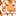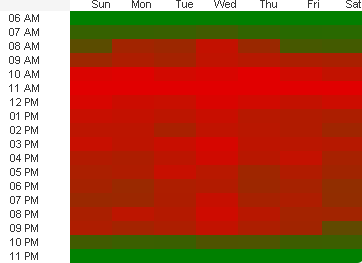# QlikView App Dev

Discussion Board for collaboration related to QlikView App Development.

Announcements
cancel
Showing results for
Did you mean:Creator

## Table as heat map not colored (with colormix) as expected

Hi,

I'm trying to create a heatmap using a pivot table but it isnt showing the colors as expected, and cant find any configuration that can help me.

This is the way I want  the table to be shown. The weekday dim should be the rows and the time dim the columns.Unfortunatly,  the colormix used in the expression background is working vertically instead of horizontally, which is what I need.

As you can see below, if I switch the dims, the colormix does what I need but unfortunatly my client wants to see the table the other way.Has anyone found a way to solve this ?

Best regards,

Diego

1 Solution

Accepted SolutionsMVP

Try this

```Aggr(
ColorMix1 ((1+Sign(2*(COUNT( DISTINCT [Transaction #] )-RangeMin (top(total COUNT( DISTINCT [Transaction #] ),1,NoOfRows(total))))/(RangeMax (top(total COUNT( DISTINCT  [Transaction #] ),1,NoOfRows(total)))-RangeMin (top(total COUNT( DISTINCT  [Transaction #] ),1,NoOfRows(total))))-1)*Sqrt(Fabs((2*(COUNT( DISTINCT  [Transaction #] )-RangeMin (top(total COUNT( DISTINCT  [Transaction #] ),1,NoOfRows(total))))/(RangeMax (top(total COUNT( DISTINCT  [Transaction #] ),1,NoOfRows(total)))-RangeMin (top(total COUNT( DISTINCT  [Transaction #] ),1,NoOfRows(total))))-1))))/2, ARGB(255, 0, 128, 0), ARGB(255, 225, 0, 0))
, WeekDay, Time)```
4 RepliesMVP
What exactly is your ColorMix expression?Creator
Author

This is what QV generated:

ColorMix1 ((1+Sign(2*(COUNT( DISTINCT [Transaction #] )-RangeMin (top(total COUNT( DISTINCT [Transaction #] ),1,NoOfRows(total))))/(RangeMax (top(total COUNT( DISTINCT  [Transaction #] ),1,NoOfRows(total)))-RangeMin (top(total COUNT( DISTINCT  [Transaction #] ),1,NoOfRows(total))))-1)*Sqrt(Fabs((2*(COUNT( DISTINCT  [Transaction #] )-RangeMin (top(total COUNT( DISTINCT  [Transaction #] ),1,NoOfRows(total))))/(RangeMax (top(total COUNT( DISTINCT  [Transaction #] ),1,NoOfRows(total)))-RangeMin (top(total COUNT( DISTINCT  [Transaction #] ),1,NoOfRows(total))))-1))))/2, ARGB(255, 0, 128, 0), ARGB(255, 225, 0, 0))MVP

Try this

```Aggr(
ColorMix1 ((1+Sign(2*(COUNT( DISTINCT [Transaction #] )-RangeMin (top(total COUNT( DISTINCT [Transaction #] ),1,NoOfRows(total))))/(RangeMax (top(total COUNT( DISTINCT  [Transaction #] ),1,NoOfRows(total)))-RangeMin (top(total COUNT( DISTINCT  [Transaction #] ),1,NoOfRows(total))))-1)*Sqrt(Fabs((2*(COUNT( DISTINCT  [Transaction #] )-RangeMin (top(total COUNT( DISTINCT  [Transaction #] ),1,NoOfRows(total))))/(RangeMax (top(total COUNT( DISTINCT  [Transaction #] ),1,NoOfRows(total)))-RangeMin (top(total COUNT( DISTINCT  [Transaction #] ),1,NoOfRows(total))))-1))))/2, ARGB(255, 0, 128, 0), ARGB(255, 225, 0, 0))
, WeekDay, Time)```Creator
Author
It worked, thanks !